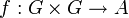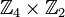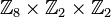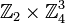# Group of cyclicity-preserving 2-cocycles for trivial group action is naturally identified with group of homomorphisms from a particular abelian group

## Statement

Suppose$G$ is a group. Then, there exists an abelian group$K$ such that, for any abelian group$A$, the group of cyclicity-preserving 2-cocycles for the trivial group action$\! f:G \times G \to A$ can be identified with the group of homomorphisms$\operatorname{Hom}(K,A)$ under pointwise addition.

## Examples

### Extreme examples

If$G$ is a cyclic group or more generally a locally cyclic group, the corresponding group$K$ is a trivial group.

### Examples of 2-groups

When$G$ is a 2-group,$K$ is also a 2-group.

Group$G$ Order Prime-base logarithm of order Group$K$ that acts as source of homomorphisms Order Prime-base logarithm of order
Klein four-group 4 2 cyclic group:Z4 4 2
direct product of Z4 and Z2 8 3 direct product of Z4 and Z2 ($\! \mathbb{Z}_4 \times \mathbb{Z}_2$) 8 3
dihedral group:D8 8 3 direct product of Z8 and V4 ($\! \mathbb{Z}_8 \times \mathbb{Z}_2 \times \mathbb{Z}_2$) 32 5
quaternion group 8 3 cyclic group:Z4 4 2
elementary abelian group:E8 8 3 direct product of Z4 and Z4 and Z4 and Z2 ($\! \mathbb{Z}_2 \times \mathbb{Z}_4^3$) 128 7
direct product of Z4 and Z4 16 4 direct product of E16 and Z8 ($\! \mathbb{Z}_2^4 \times \mathbb{Z}_8$) 128 7
SmallGroup(16,3) 16 4$\! \mathbb{Z}_2^5 \times \mathbb{Z}_4 \times \mathbb{Z}_8$ 1024 10
nontrivial semidirect product of Z4 and Z4 16 4 direct product of E16 and Z8 ($\! \mathbb{Z}_2^4 \times \mathbb{Z}_8$) 128 7
direct product of Z8 and Z2 16 4 direct product of Z4 and V4 ($\! \mathbb{Z}_2^2 \times \mathbb{Z}_4$) 16 4
M16 16 4 direct product of Z4 and V4 ($\! \mathbb{Z}_2^2 \times \mathbb{Z}_4$) 16 4
dihedral group:D16 16 4$\mathbb{Z}_2^6 \times \mathbb{Z}_{16}$ 1024 10
semidihedral group:SD16 16 4 direct product of E16 and Z8 ($\! \mathbb{Z}_2^4 \times \mathbb{Z}_8$) 128 7
generalized quaternion group:Q16 16 4 direct product of Z8 and V4 ($\! \mathbb{Z}_2^2 \times \mathbb{Z}_8$) 32 5
direct product of Z4 and V4 16 4$\! \mathbb{Z}_2^4 \times \mathbb{Z}_4^3$ 1024 10
direct product of D8 and Z2 16 4$\! \mathbb{Z}_2^6 \times \mathbb{Z}_4^2 \times \mathbb{Z}_8$ 8192 13
direct product of Q8 and Z2 16 4$\! \mathbb{Z}_2^2 \times \mathbb{Z}_4^3$ 256 8
central product of D8 and Z4 16 4$\! \mathbb{Z}_2^4 \times \mathbb{Z}_4^3$ 1024 10
elementary abelian group:E16 16 4$\! \mathbb{Z}_2^5 \times \mathbb{Z}_4^6$ 131072 17
elementary abelian group:E32 32 5$\! \mathbb{Z}_2^{16} \times \mathbb{Z}_4^{10}$ 68719476736 32

### Examples of other p-groups

We consider the case$\! p = 3$.

Group$G$ Order Prime-base logarithm of order Group$K$ that acts as source of homomorphisms Order Prime-base logarithm of order
elementary abelian group:E9 9 2 elementary abelian group:E27 27 3
direct product of Z9 and Z3 27 3 elementary abelian group:E243 243 5